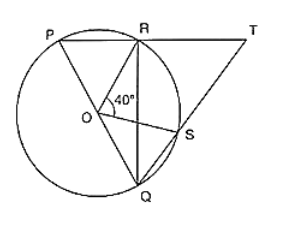# In figure, O is the centre of a circle and PQ is a diameter.

Question:

In figure, O is the centre of a circle and PQ is a diameter. If

∠ROS = 40°, find ∠RTS.Solution:

Since PQ is diameter

Then,

∠PRQ = 90° [Angle in semicircle]

∴ ∠PRQ + ∠TRQ = 180° [Linear pair of angle]

900 + ∠TRQ = 180°

∠TRQ = 180° − 90° = 90°.

By degree measure theorem

∠ROS = 2∠RQS

⇒ 40° = 2∠RQS

⇒ ∠RQS = 40°/2 = 20°

In ΔRQT, by Angle sum property

∠RQT + ∠QRT + ∠RTS = 180°

⇒ 20° + 90° + ∠RTS = 180°

⇒ ∠RTS = 180° − 20° − 90° = 70°